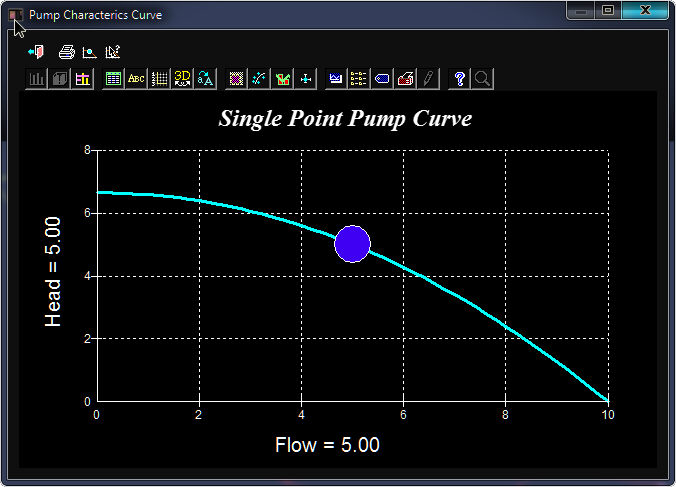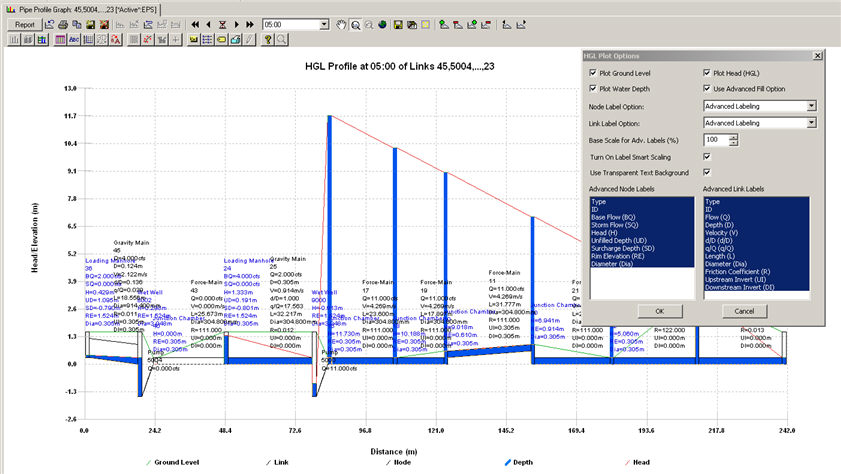#INFOSEWER

# Pumps in InfoSewer and H20Map Sewer

Pumps in InfoSewer and H20Map Sewer

A pump is a link that imparts energy to a fluid thereby raising its hydraulic head. Pumps are needed in a gravity sewer system to prevent deep excavations, and are designed to lift the fluid to a desired level that produces gravity flow condition further downstream.

The relationship describing the head gained to a fluid as a function of its flow rate through the pump is defined as the pump characteristic curve.InfoSewerH20Map Sewer represents pumps as links of negligible length which are directly connected to wet-wells. The principal input parameters for a pump are:

· The wet-well ID representing the inlet side of the pump

· The manhole junction chamber ID representing the discharge side of the pump

· The additional number of parallel (identical) pumps with the same characteristics (optional)

InfoSewerH20Map Sewer uses different types of pump curves depending on the number of head-flow data points supplied. Three different types of pumps can be modeled as follows:

· Fixed Capacity representing a fixed pump flow. The pump flow is set to the fixed capacity and is independent of the flow entering the wet-well. It is assumed that the flow will transfer at the fixed rate, independent of the head requirements.

· Single-Point Curve – A single-point pump curve is defined by a single head-flow data point describing the desired pump operating point (design point).InfoSewerH20Map Sewer assumes that the cutoff head (at zero flow) is 133% of the design head and the maximum flow (at zero head) is twice the design flow. It then treats the curve as a three-point curve.

· Three-Point Curve – A three-point pump curve is defined by three points of operating data. InfoSewerH20Map Sewer will fit a continuous function of the form:By supplying InfoSewerH20Map Sewer with the cutoff head Ho and two other points [(H1,
Q1), (H2, Q2)], the program is able to estimate values for a and b from:The computed results for pumps are the flow pumped and the head gained.

For variable speed pumps, the pump curve shifts as the speed changes. The relationships between flow (Q) and head (H) at speeds n1 and n2 areBy definition, the original pump curve supplied to the program has a relative speed setting of 1. If the pump speed doubles, then the relative setting would be 2; if run at half speed, the relative setting is 0.5 and so on. The figure below illustrates how changing a pump’s speed setting affects its characteristic curve.If n denotes the pump speed ratio (n1/n2), then the pump characteristic curve becomes:From H2OCalc a section on Pump Inertia

Pump Inertia

The pump inertia dialog box is shown below. Click here for the methodology.## Input for pump inertia:

Flow Unit – Select the desired flow unit.

Pump Speed – Pump speed (number of revolutions per unit time).

Discharge – Rated pump flow rate.

Pump Head – Rated pump head corresponding to the rated pump discharge.

Efficiency – Pump efficiency (ratio of power output to power input).

Pump Power – Power computed from pump head, pump flow and pump efficiency.

## Output for pump inertia:

Fairly Old Pump – Pump inertia computed assuming that the pump is fairly old.

Small, Light Weigh Pump – Pump inertial computed assuming that the pump is small and light weight.

Motor Inertia – Inertia of the motor.

Specific Speed – Specific speed of the pump.

Note: The total (or combined) inertia of a pump is the sum of the pump inertia and the motor inertia. The pump inertia is the momentum of inertia of pump impeller and entrained liquid, while the th motor inertia is the momentum of inertia of motor rotor, shaft, and couplings. For transient analysis, the total inertia should be used to properly describe the pump’s resistance to change in momentum. The higher its inertia, the longer it will take the pump to stop spinning after a shutdown. Pump inertia can be increased using a flywheel (may not be effective for very long pipes).